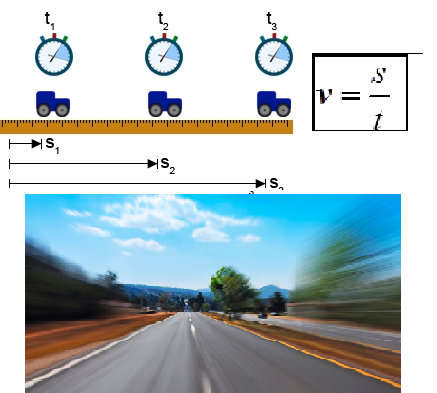The simplest example of straight linear motion is the straight line movement at constant speed. A linear movement is a movement where the body travels equal lengths during the same amounts of time.

Example: A car has passed the lengths of the road of 8m during the same time interval of 2s. From the above example, we can conclude that the speed is constant, and in two seconds the car moves by 8 meters, which means that for during 1 second the car moves 4 meters, and its speed is 4m/s,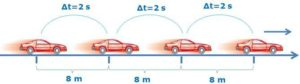v = const.

When the body travels 2 meters in 1 second, we say its speed is 2 meters per second.This expression is obtained when we divide the length of the traveled path with the time needed for the travel.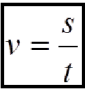The speed of the straight linear motion is equal to the traveled path in the unit of time. The speed unit in SI is a meter per second. The body moves at a speed of 1 m / s if it travels 1 meter of the path every second.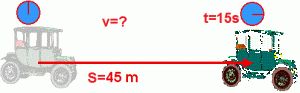Example: Car’s speed after 15 seconds, which has crossed the path of 45 meters, is v = s / t => v = 45m / 15s; v = 3m / s

We can calculate the speed if we know the relationship between time and length of the traveled path. From the given relation we can calculate the time of movement if the traveled path and the velocity are known: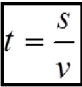Example: The picture shows the car traveled 12m with the speed of 8m / s.  We need to find the time of the car’s movement. When we divide the length of the traveled path with the speed, we get that the time for which the car has crossed the path is 1.5s.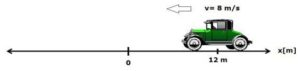If we know the speed and the time, we can also calculate the traveled path.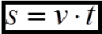Any of the three quantities that determine the motion can be calculated if the other two are known to us. The speed can also often measured in kilometers per hour (km / h) and kilometers per second (km / s).

The value of the speed in meters per second for some examples:Nails grow at a speed:

0,000 000 001 m/sThe snail is moving at a speed of:

0.000 002 m/s.Pedestrian is moving at a speed of:

1.5 m/s.Man runs at a speed of:

10 m/s.A fast train runs at a speed of:

80 m/s.A plane flies at a speed of

250 m / s.Sound travels at:

340 m/sLight travels at:

300 000 000 m/s.

SHARE
Previous articleKirchhoff’s rules
Next articleThe Surface Area and the Volume